Geek Repo

Github PK Tool

# 使用循环神经网络破解验证码

captcha 部分的代码和之前卷积神经网络识别的一样，只是将 `n_class` 改为了 `len(characters)+1`，因为我们需要添加一个空白类用于 CTC Loss。

```from captcha.image import ImageCaptcha
import matplotlib.pyplot as plt
import numpy as np
import random

%matplotlib inline
%config InlineBackend.figure_format = 'retina'

import string
characters = string.digits + string.ascii_uppercase
print(characters)

width, height, n_len, n_class = 170, 80, 4, len(characters)+1

random_str = ''.join([random.choice(characters) for j in range(4)])
img = generator.generate_image(random_str)

plt.imshow(img)
plt.title(random_str)```

## CTC Loss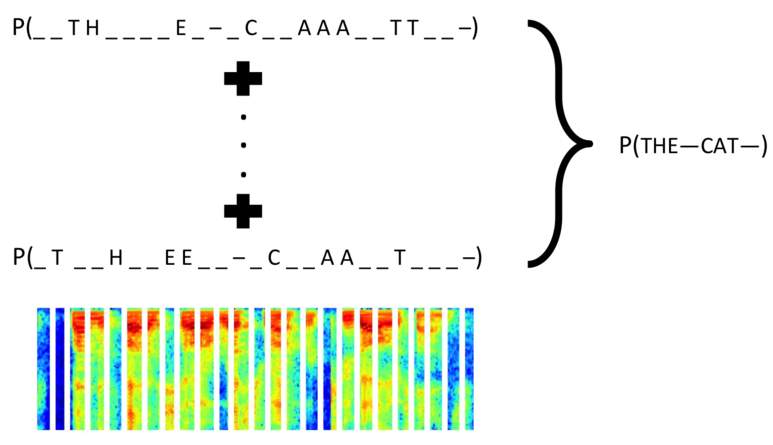• y_pred 是模型的输出，是按顺序输出的37个字符的概率，因为我们这里用到了循环神经网络，所以需要一个空白字符的类；
• labels 是验证码，是四个数字，每个数字对应字符的编号；
• input_length 表示 y_pred 的长度，我们这里是15；
• label_length 表示 labels 的长度，我们这里是4。
```from keras import backend as K

def ctc_lambda_func(args):
y_pred, labels, input_length, label_length = args
y_pred = y_pred[:, 2:, :]
return K.ctc_batch_cost(labels, y_pred, input_length, label_length)```

## 模型结构

```from keras.models import *
from keras.layers import *
from keras.optimizers import *
rnn_size = 128

input_tensor = Input((width, height, 3))
x = input_tensor
x = Lambda(lambda x:(x-127.5)/127.5)(x)
for i in range(3):
for j in range(2):
x = Convolution2D(32*2**i, 3, kernel_initializer='he_uniform')(x)
x = BatchNormalization()(x)
x = Activation('relu')(x)
x = MaxPooling2D((2, 2))(x)

conv_shape = x.get_shape().as_list()
rnn_length = conv_shape
rnn_dimen = conv_shape*conv_shape
print(conv_shape, rnn_length, rnn_dimen)
x = Reshape(target_shape=(rnn_length, rnn_dimen))(x)
rnn_length -= 2

x = Dense(rnn_size, kernel_initializer='he_uniform')(x)
x = BatchNormalization()(x)
x = Activation('relu')(x)
x = Dropout(0.2)(x)

gru_1 = GRU(rnn_size, return_sequences=True, kernel_initializer='he_uniform', name='gru1')(x)
gru_1b = GRU(rnn_size, return_sequences=True, kernel_initializer='he_uniform',
go_backwards=True, name='gru1_b')(x)

gru_2 = GRU(rnn_size, return_sequences=True, kernel_initializer='he_uniform', name='gru2')(x)
gru_2b = GRU(rnn_size, return_sequences=True, kernel_initializer='he_uniform',
go_backwards=True, name='gru2_b')(x)
x = concatenate([gru_2, gru_2b])

x = Dropout(0.2)(x)
x = Dense(n_class, activation='softmax')(x)
base_model = Model(inputs=input_tensor, outputs=x)

labels = Input(name='the_labels', shape=[n_len], dtype='float32')
input_length = Input(name='input_length', shape=, dtype='int64')
label_length = Input(name='label_length', shape=, dtype='int64')
loss_out = Lambda(ctc_lambda_func, output_shape=(1,),
name='ctc')([x, labels, input_length, label_length])

model = Model(inputs=[input_tensor, labels, input_length, label_length], outputs=[loss_out])
model.compile(loss={'ctc': lambda y_true, y_pred: y_pred}, optimizer='adam')```

## 模型可视化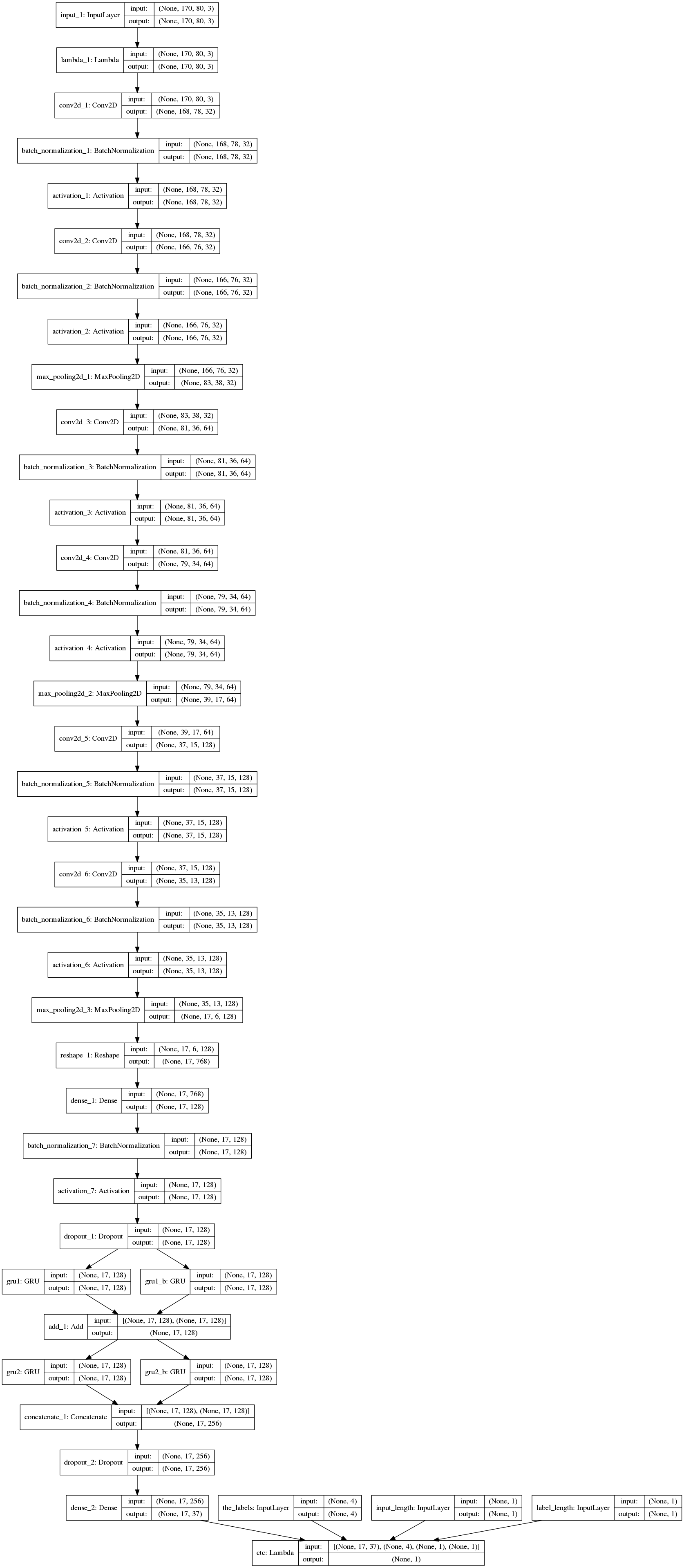## 数据生成器

• X 是一批图片；
• y 是每个图片对应的 label，最大长度为 n_len；
• input_length 表示模型输出的长度，我们这里是15；
• label_length 表示 labels 的长度，我们这里是4。

```def gen(batch_size=128):
X = np.zeros((batch_size, width, height, 3), dtype=np.uint8)
y = np.zeros((batch_size, n_len), dtype=np.uint8)
while True:
for i in range(batch_size):
random_str = ''.join([random.choice(characters) for j in range(n_len)])
X[i] = np.array(generator.generate_image(random_str)).transpose(1, 0, 2)
y[i] = [characters.find(x) for x in random_str]
yield [X, y, np.ones(batch_size)*rnn_length, np.ones(batch_size)*n_len], np.ones(batch_size)```

```(X_vis, y_vis, input_length_vis, label_length_vis), _ = next(gen(1))
print(X_vis.shape, y_vis, input_length_vis, label_length_vis)

plt.imshow(X_vis.transpose(1, 0, 2))
plt.title(''.join([characters[i] for i in y_vis]))```

`(1, 170, 80, 3) [[29 4 21 21]] [ 15.] [ 4.]`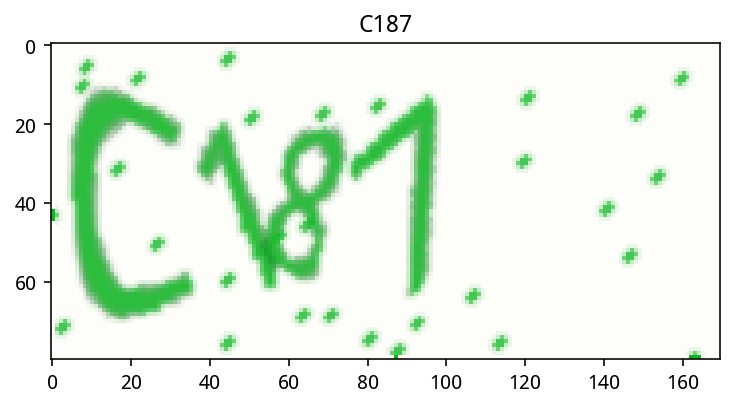• X 的 shape 是 `(1, 170, 80, 3)`，如果有 n 张图，shape 就是 `(n, 170, 80, 3)`
• y 是 label，我们可以看到生成的图片是 T4LL，那么按上面的 characters，label 就是 `[29 4 21 21]`，外面还有一个框是因为这里面可以有 n 个 label
• input_length 表示模型输出的长度，我们这里是15；
• label_length 表示 labels 的长度，我们这里是4。

## 评估模型

```def evaluate(batch_size=128, steps=10):
batch_acc = 0
generator = gen(batch_size)
for i in range(steps):
[X_test, y_test, _, _], _  = next(generator)
y_pred = base_model.predict(X_test)
shape = y_pred[:,2:,:].shape
ctc_decode = K.ctc_decode(y_pred[:,2:,:], input_length=np.ones(shape)*shape)
out = K.get_value(ctc_decode)[:, :n_len]
if out.shape == n_len:
batch_acc += (y_test == out).all(axis=1).mean()
return batch_acc / steps```

## 评估回调

```from keras.callbacks import *

class Evaluator(Callback):
def __init__(self):
self.accs = []

def on_epoch_end(self, epoch, logs=None):
acc = evaluate(steps=20)*100
self.accs.append(acc)
print('')
print('acc: %f%%' % acc)

evaluator = Evaluator()```

## 训练模型

```h = model.fit_generator(gen(128), steps_per_epoch=400, epochs=20,
callbacks=[evaluator],
validation_data=gen(128), validation_steps=20)```
```model.compile(loss={'ctc': lambda y_true, y_pred: y_pred}, optimizer=Adam(1e-4))
h2 = model.fit_generator(gen(128), steps_per_epoch=400, epochs=20,
callbacks=[evaluator],
validation_data=gen(128), validation_steps=20)```

```plt.figure(figsize=(10, 4))
plt.subplot(1, 2, 1)
plt.plot(h.history['loss'] + h2.history['loss'])
plt.plot(h.history['val_loss'] + h2.history['val_loss'])
plt.legend(['loss', 'val_loss'])
plt.ylabel('loss')
plt.xlabel('epoch')
plt.ylim(0, 1)

plt.subplot(1, 2, 2)
plt.plot(evaluator.accs)
plt.ylabel('acc')
plt.xlabel('epoch')```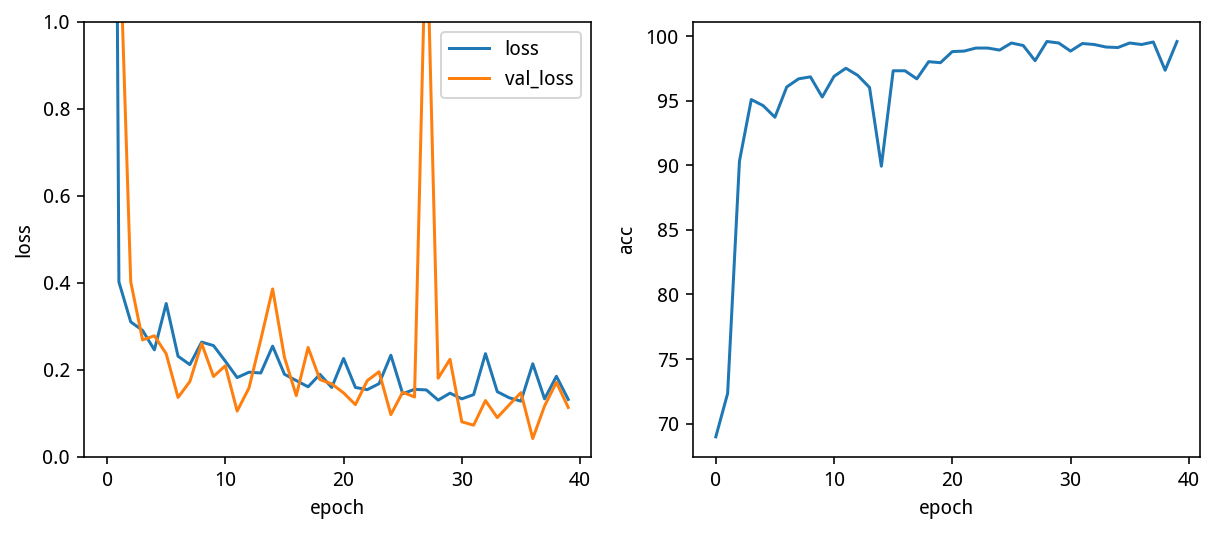``````Epoch 20/20
399/400 [============================>.] - ETA: 0s - loss: 0.1593
acc: 97.929688%
400/400 [==============================] - 122s - loss: 0.1589 - val_loss: 0.1671
``````

``````Epoch 20/20
399/400 [============================>.] - ETA: 0s - loss: 0.1317
acc: 99.570312%
400/400 [==============================] - 123s - loss: 0.1315 - val_loss: 0.1130
``````

## 测试模型

```(X_vis, y_vis, input_length_vis, label_length_vis), _ = next(gen(12))

y_pred = base_model.predict(X_vis)
shape = y_pred[:,2:,:].shape
ctc_decode = K.ctc_decode(y_pred[:,2:,:], input_length=np.ones(shape)*shape)
out = K.get_value(ctc_decode)[:, :4]

plt.figure(figsize=(16, 8))
for i in range(12):
plt.subplot(3, 4, i+1)
plt.imshow(X_vis[i].transpose(1, 0, 2))
plt.title('pred:%s\nreal :%s' % (''.join([characters[x] for x in out[i]]),
''.join([characters[x] for x in y_vis[i]])))```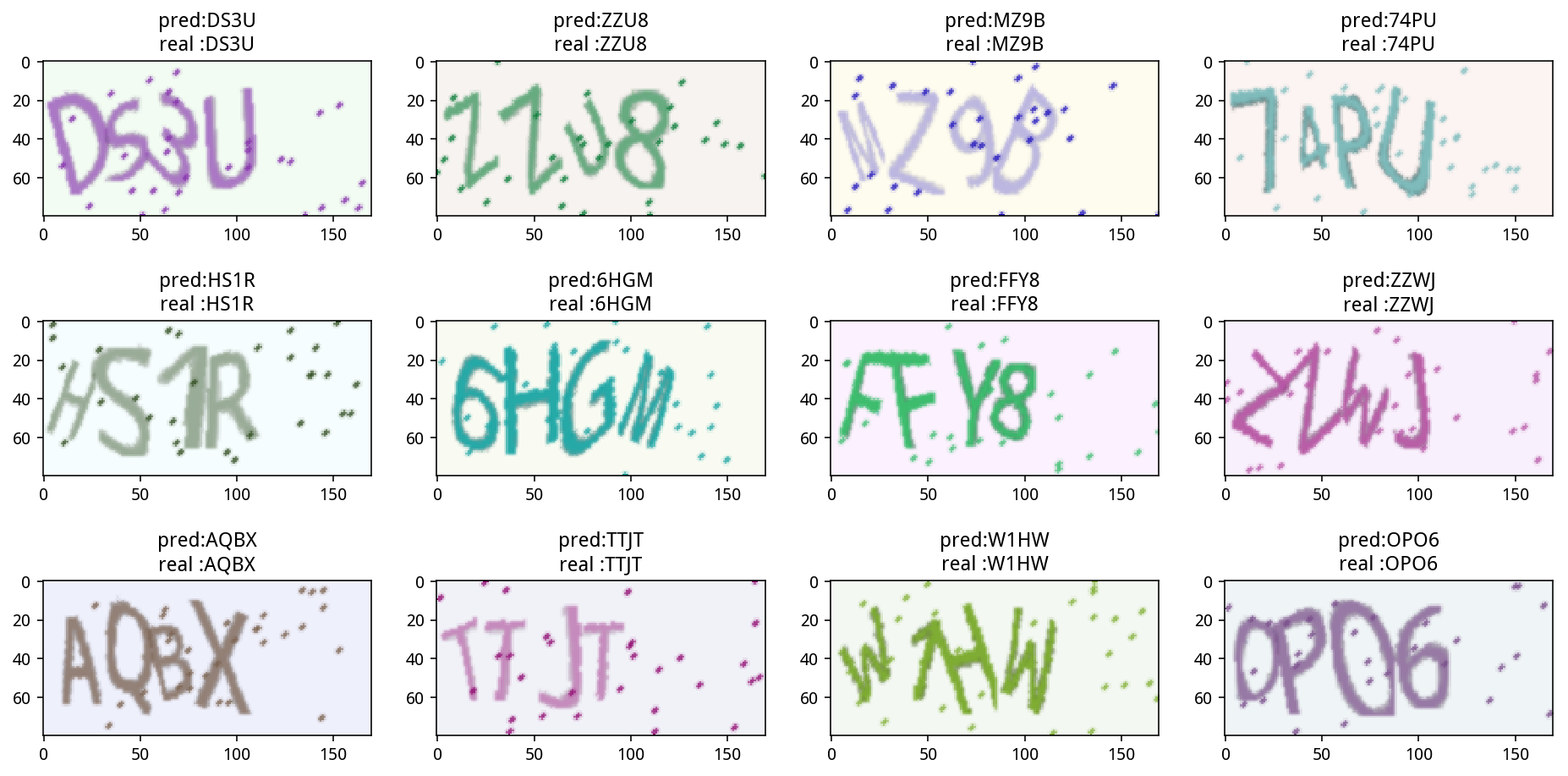## 评估模型

```(X_vis, y_vis, input_length_vis, label_length_vis), _ = next(gen(10000))

y_pred = base_model.predict(X_vis, verbose=1)
shape = y_pred[:,2:,:].shape
ctc_decode = K.ctc_decode(y_pred[:,2:,:], input_length=np.ones(shape)*shape)
out = K.get_value(ctc_decode)[:, :4]

(y_vis == out).all(axis=1).mean()

# 0.99460000000000004```

```from collections import Counter
Counter(''.join([characters[i] for i in y_vis[y_vis != out]]))

Counter({'0': 37, 'O': 14, 'Q': 1, 'T': 1, 'W': 1})```

```characters2 = characters + ' '

random_str = '0O0O'
X_test = np.array(generator.generate_image(random_str))
X_test = X_test.transpose(1, 0, 2)
X_test = np.expand_dims(X_test, 0)

y_pred = base_model.predict(X_test)
shape = y_pred[:,2:,:].shape
ctc_decode = K.ctc_decode(y_pred[:,2:,:], input_length=np.ones(shape)*shape)
out = K.get_value(ctc_decode)[:, :4]
out = ''.join([characters[x] for x in out])

plt.imshow(X_test.transpose(1, 0, 2))
plt.title('pred:' + str(out))

argmax = np.argmax(y_pred, axis=2)
list(zip(argmax, ''.join([characters2[x] for x in argmax])))```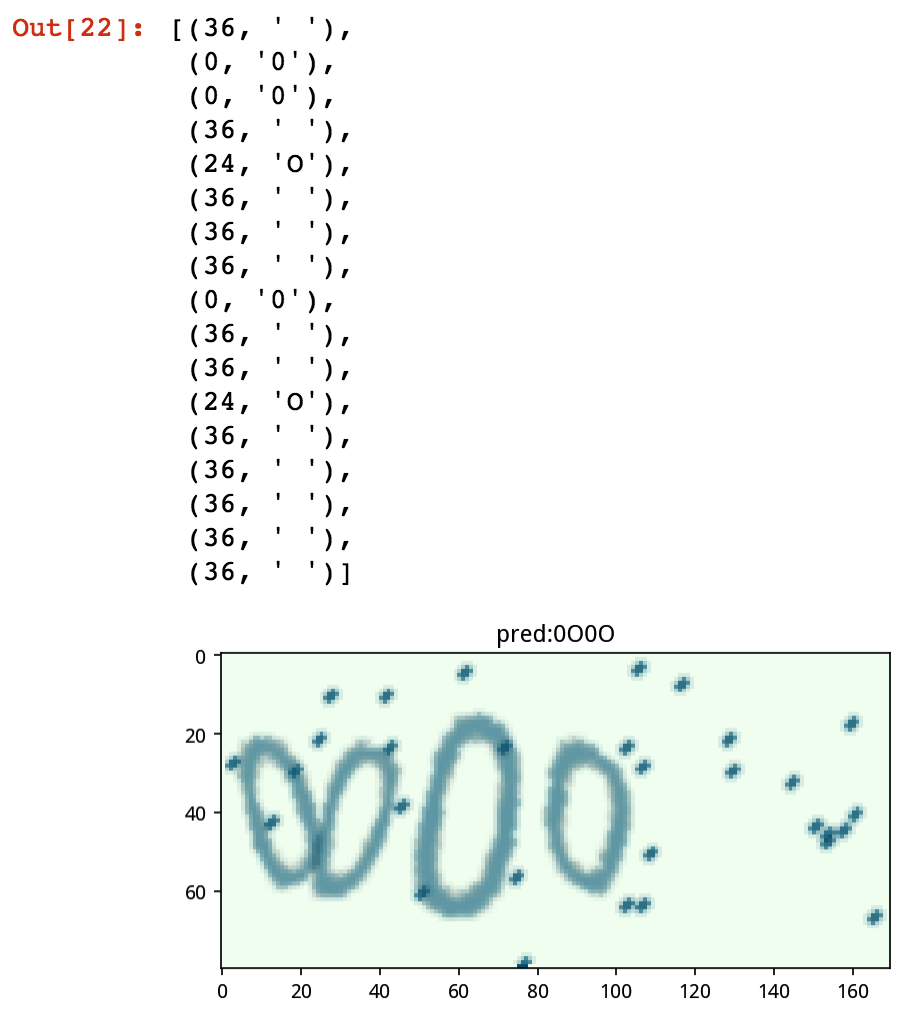# 四则混合运算识别（初赛）

## 问题描述

### 数据集

3个运算数：3个0到9的整型数字； 2个运算符：可以是+、-、*，分别代表加法、减法、乘法 0或1对括号：括号可能是0对或者1对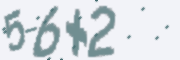``````(3-7)+5 1
5-6+2 1
(6+7)*2 26
(4+2)+7 13
(6*4)*4 96
``````

### 生成器

```import string
import random

digits = string.digits
operators = '+-*'
characters = digits + operators + '() '

def generate():
seq = ''
k = random.randint(0, 2)

if k == 1:
seq += '('
seq += random.choice(digits)
seq += random.choice(operators)
if k == 2:
seq += '('
seq += random.choice(digits)
if k == 1:
seq += ')'
seq += random.choice(operators)
seq += random.choice(digits)
if k == 2:
seq += ')'

return seq```

```import random

def generate():
ts = [u'{}{}{}{}{}', '({}{}{}){}{}', '{}{}({}{}{})']
ds = u'0123456789'
os = u'+-*'
cs = [random.choice(ds) if x%2 == 0 else random.choice(os) for x in range(5)]
return random.choice(ts).format(*cs)```

```# line 191-194
if c != '-':
im = im.resize((w2, h2))
im = im.transform((w, h), Image.QUAD, data)```

```import string
import os

digits = string.digits
operators = '+-*'
characters = digits + operators + '() '
width, height, n_len, n_class = 180, 60, 7, len(characters) + 1
font_sizes=range(35, 56),
fonts=['fonts/%s'%x for x in os.listdir('fonts') if '.tt' in x]
)
generator.generate_image('(1-2)-3')```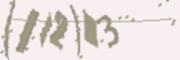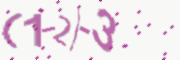## 模型结构

```from keras.layers import *
from keras.models import *
from make_parallel import make_parallel
rnn_size = 128

input_tensor = Input((width, height, 3))
x = input_tensor
for i in range(3):
x = Conv2D(32*2**i, (3, 3), kernel_initializer='he_normal')(x)
x = BatchNormalization()(x)
x = Activation('relu')(x)
x = Conv2D(32*2**i, (3, 3), kernel_initializer='he_normal')(x)
x = BatchNormalization()(x)
x = Activation('relu')(x)
x = MaxPooling2D(pool_size=(2, 2))(x)

conv_shape = x.get_shape()
x = Reshape(target_shape=(int(conv_shape), int(conv_shape*conv_shape)))(x)

x = Dense(128, kernel_initializer='he_normal')(x)
x = BatchNormalization()(x)
x = Activation('relu')(x)

gru_1 = GRU(rnn_size, return_sequences=True, kernel_initializer='he_normal', name='gru1')(x)
gru_1b = GRU(rnn_size, return_sequences=True, go_backwards=True, kernel_initializer='he_normal',
name='gru1_b')(x)

gru_2 = GRU(rnn_size, return_sequences=True, kernel_initializer='he_normal', name='gru2')(gru1_merged)
gru_2b = GRU(rnn_size, return_sequences=True, go_backwards=True, kernel_initializer='he_normal',
name='gru2_b')(gru1_merged)
x = concatenate([gru_2, gru_2b])
x = Dropout(0.25)(x)
x = Dense(n_class, kernel_initializer='he_normal', activation='softmax')(x)
base_model = Model(input=input_tensor, output=x)

base_model2 = make_parallel(base_model, 4)

labels = Input(name='the_labels', shape=[n_len], dtype='float32')
input_length = Input(name='input_length', shape=(1,), dtype='int64')
label_length = Input(name='label_length', shape=(1,), dtype='int64')
loss_out = Lambda(ctc_lambda_func, name='ctc')([base_model2.output, labels, input_length, label_length])

model = Model(inputs=(input_tensor, labels, input_length, label_length), outputs=loss_out)
model.compile(loss={'ctc': lambda y_true, y_pred: y_pred}, optimizer='adam')```

BN 层主要是为了训练加速，实验结果非常好，模型收敛快了很多。

base_model 的可视化：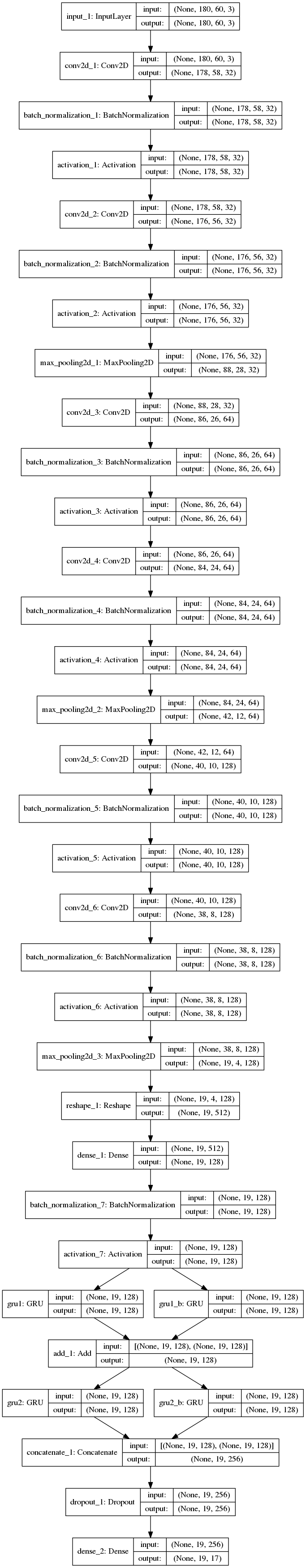model 的可视化：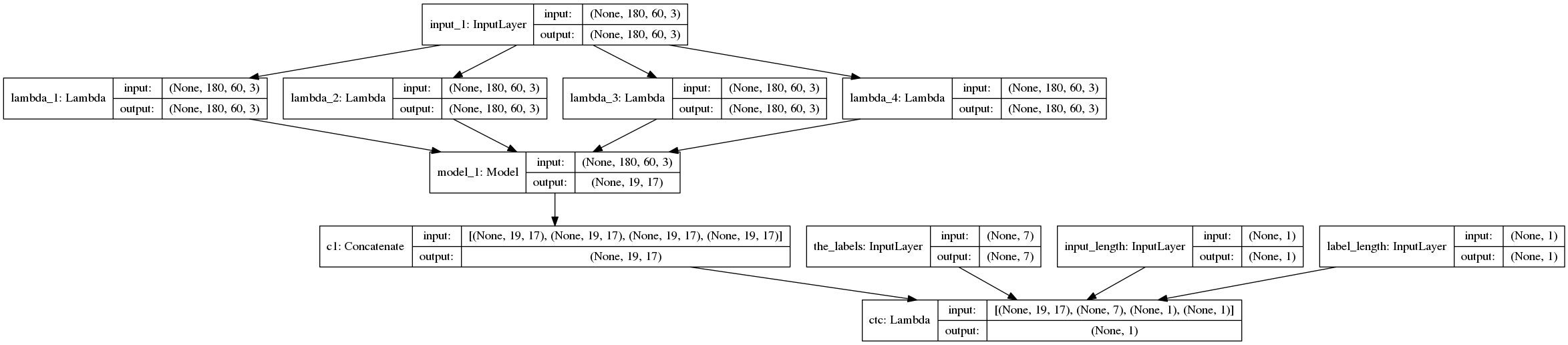## 模型训练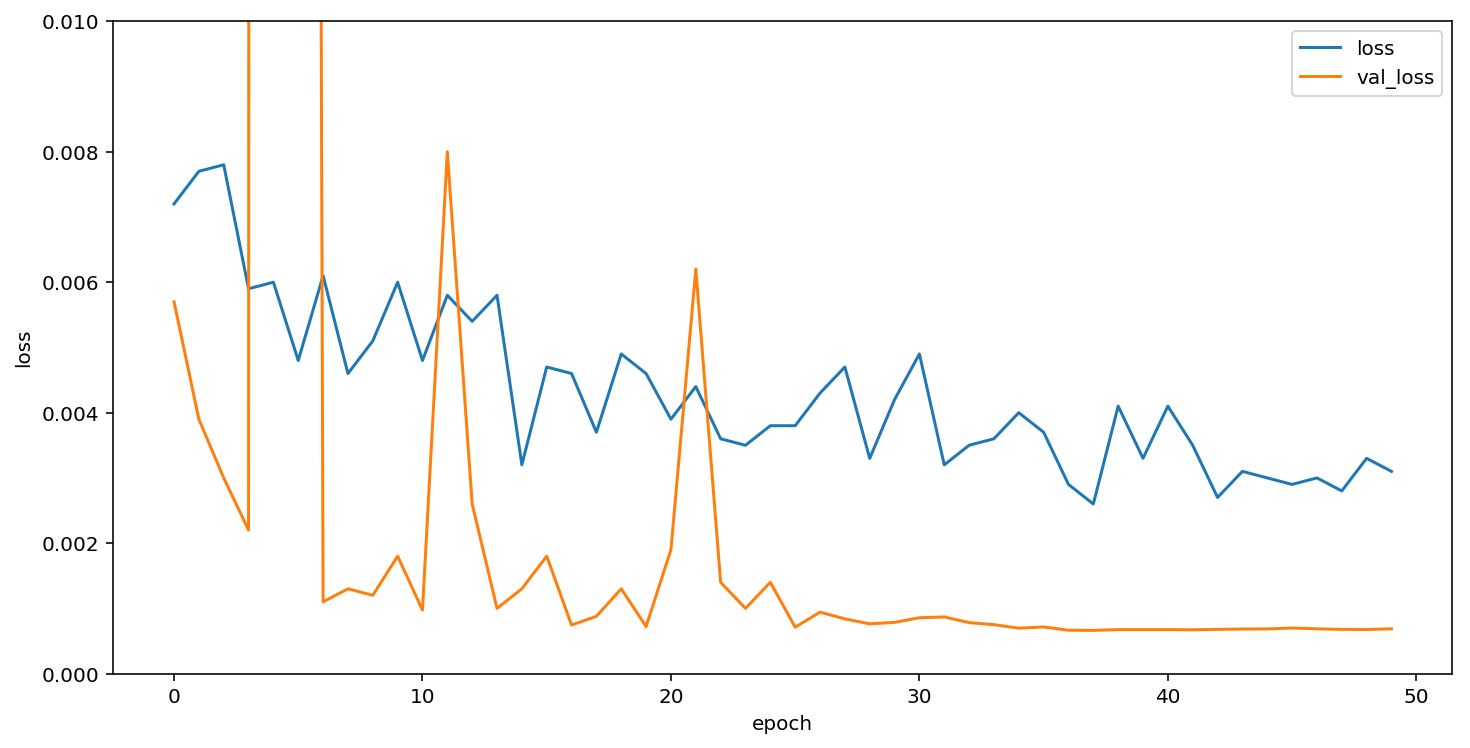## 结果可视化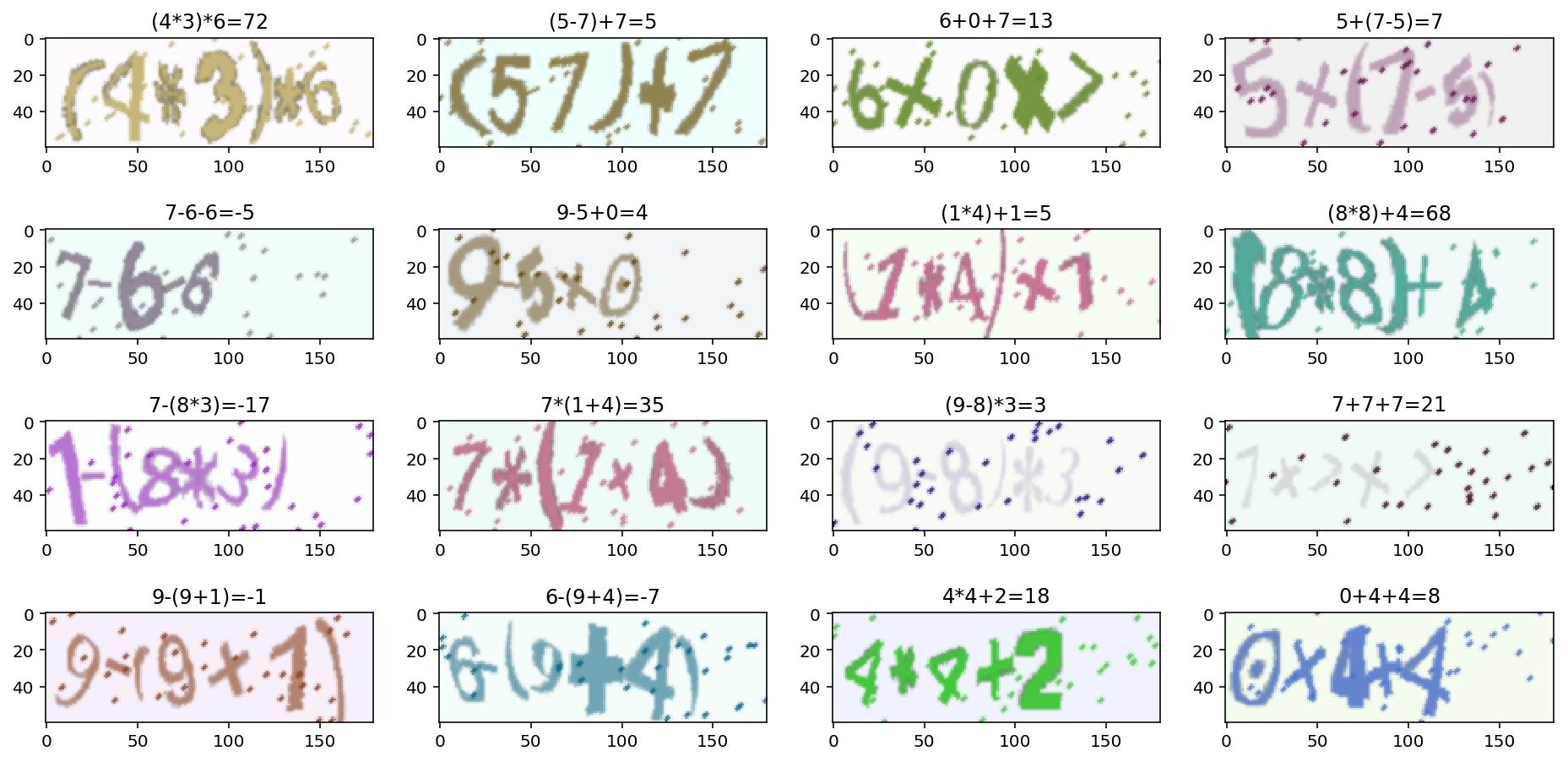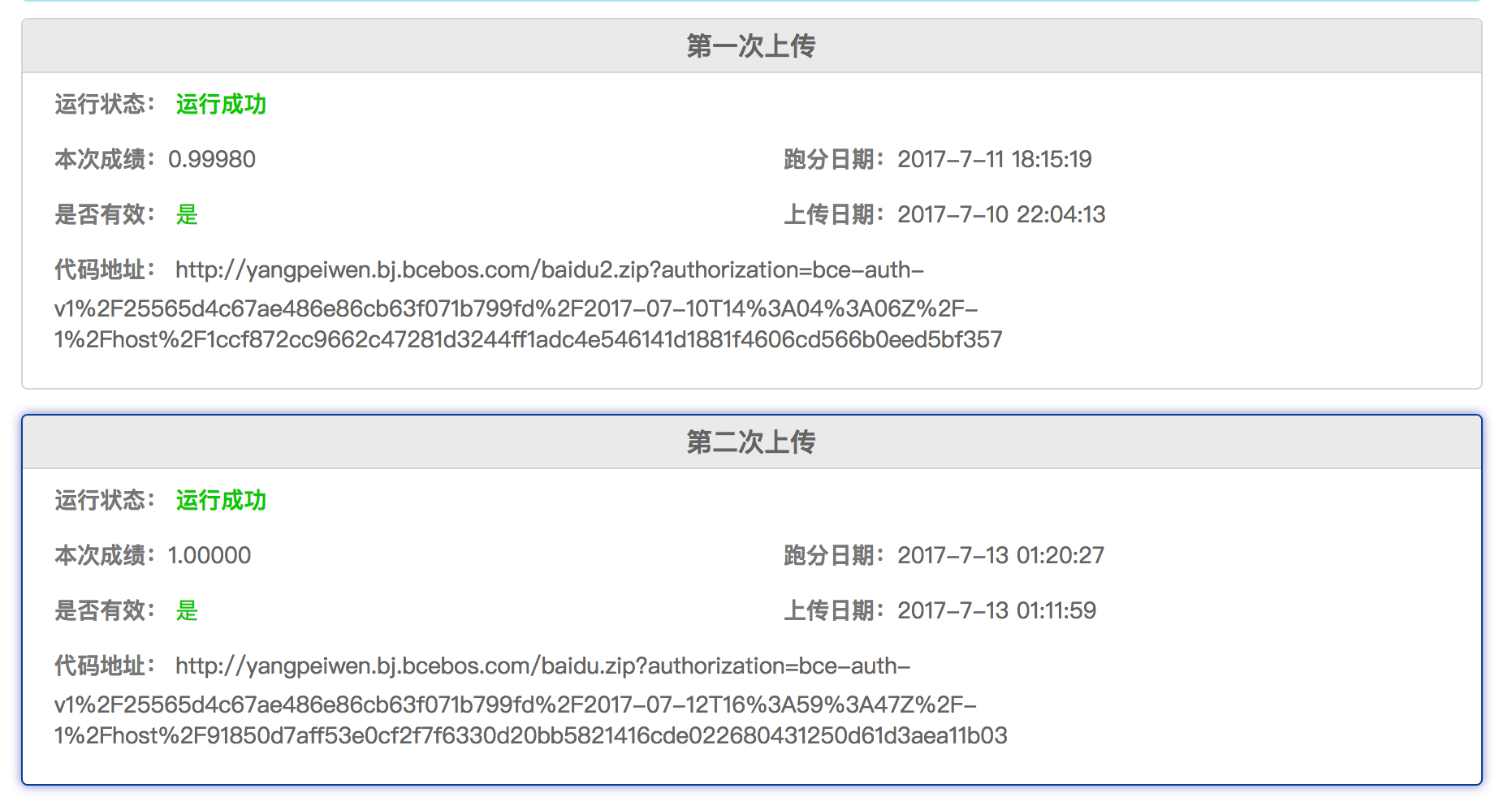## 总结

### 官方扩充测试集的难点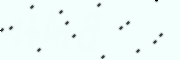```IMAGE_DIR = 'image_contest_level_1_validate'
index = 117422

img = cv2.imread('%s/%d.png' % (IMAGE_DIR, index))
gray = cv2.cvtColor(img, cv2.COLOR_BGR2GRAY)
h = cv2.equalizeHist(gray)```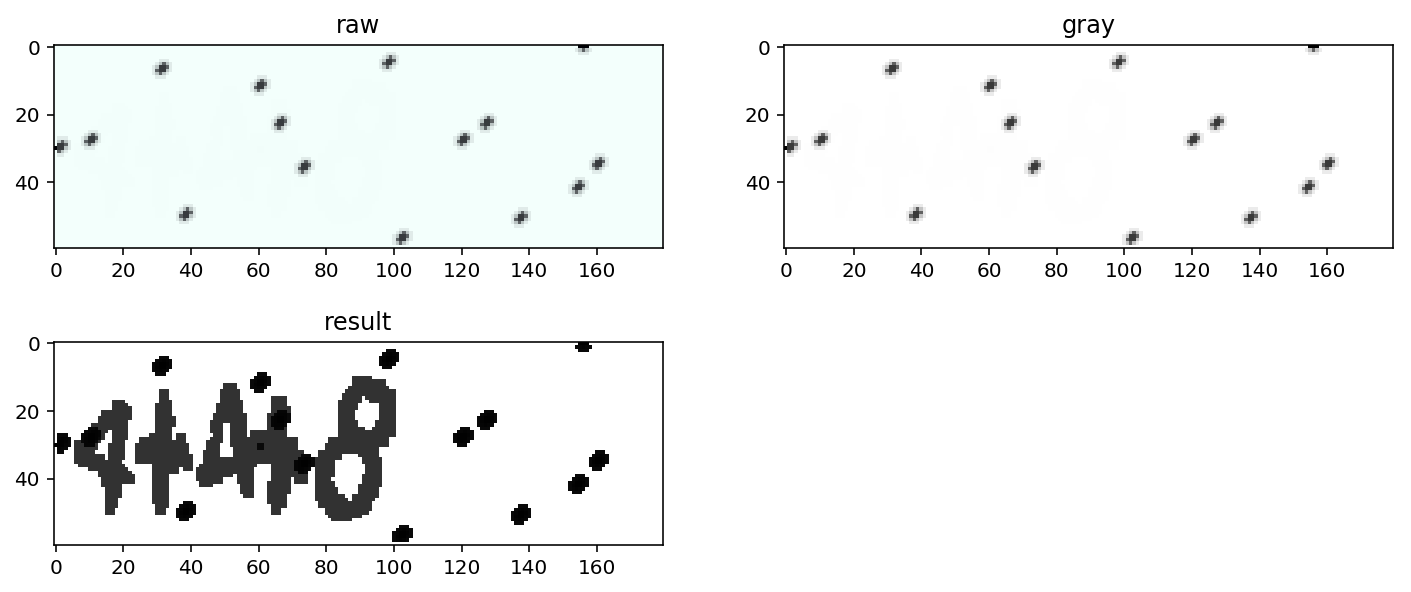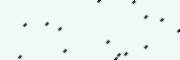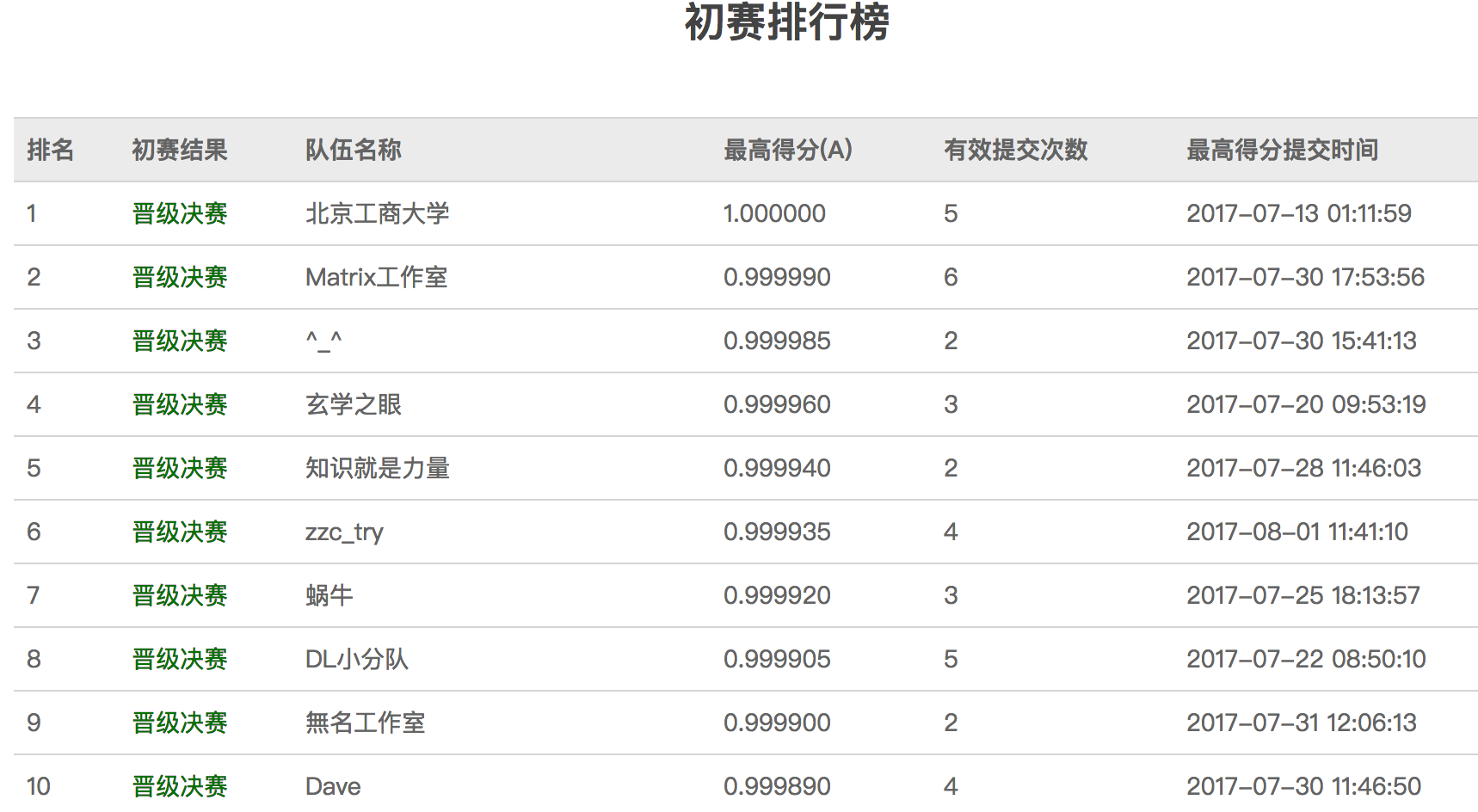# 四则混合运算识别（决赛）

## 问题描述

### 数据集

1. 图片大小不固定
2. 图片中的某一块区域为公式部分
3. 图片中包含二行或者三行的公式
4. 公式类型有两种：赋值和四则运算的公式。两行的包括由一个赋值公式和一个计算公式，三行的包括两个赋值公式和一个计算公式。加号（+） 即使旋转为 x ，仍为加号， * 是乘号
5. 赋值类的公式，变量名为一个汉字。 汉字来自两句诗（不包括逗号）： 君不见，黄河之水天上来，奔流到海不复回 烟锁池塘柳，深圳铁板烧
6. 四则运算的公式包括加法、减法、乘法、分数、括号。 其中的数字为多位数字，汉字为变量，由上面的语句赋值。
7. 输出结果的格式为：图片中的公式，一个英文空格，计算结果。 其中： 不同行公式之间使用英文分号分隔 计算结果时，分数按照浮点数计算，计算结果误差不超过0.01，视为正确。
8. 整个label文件使用UTF8编码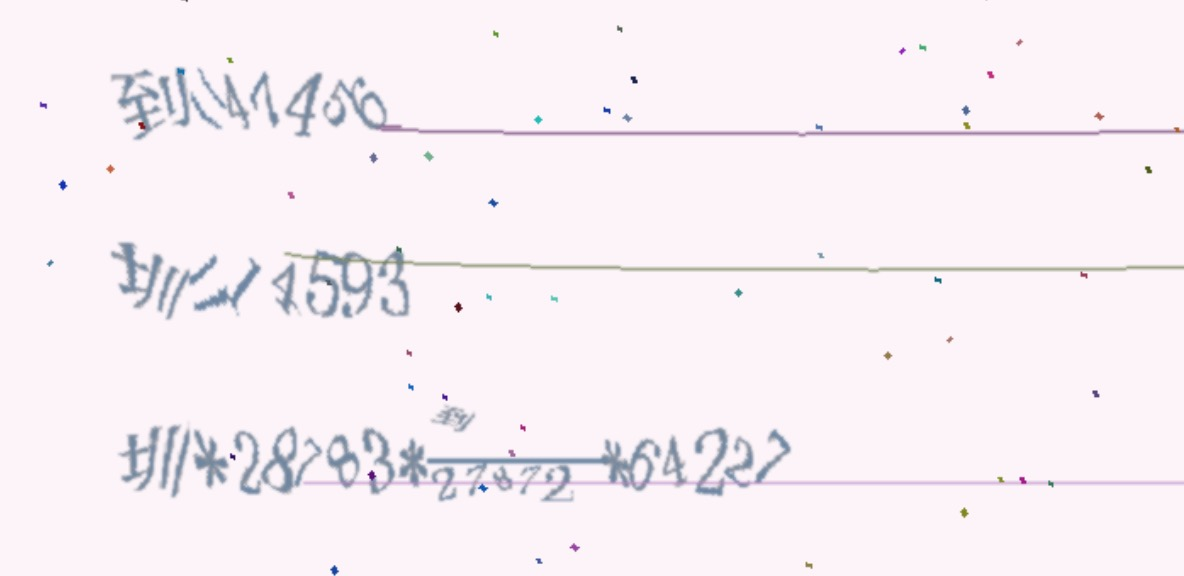## 数据的探索

### 定义

`流=42072;圳=86;(圳-(97510*45921))*流/35864`

### 分析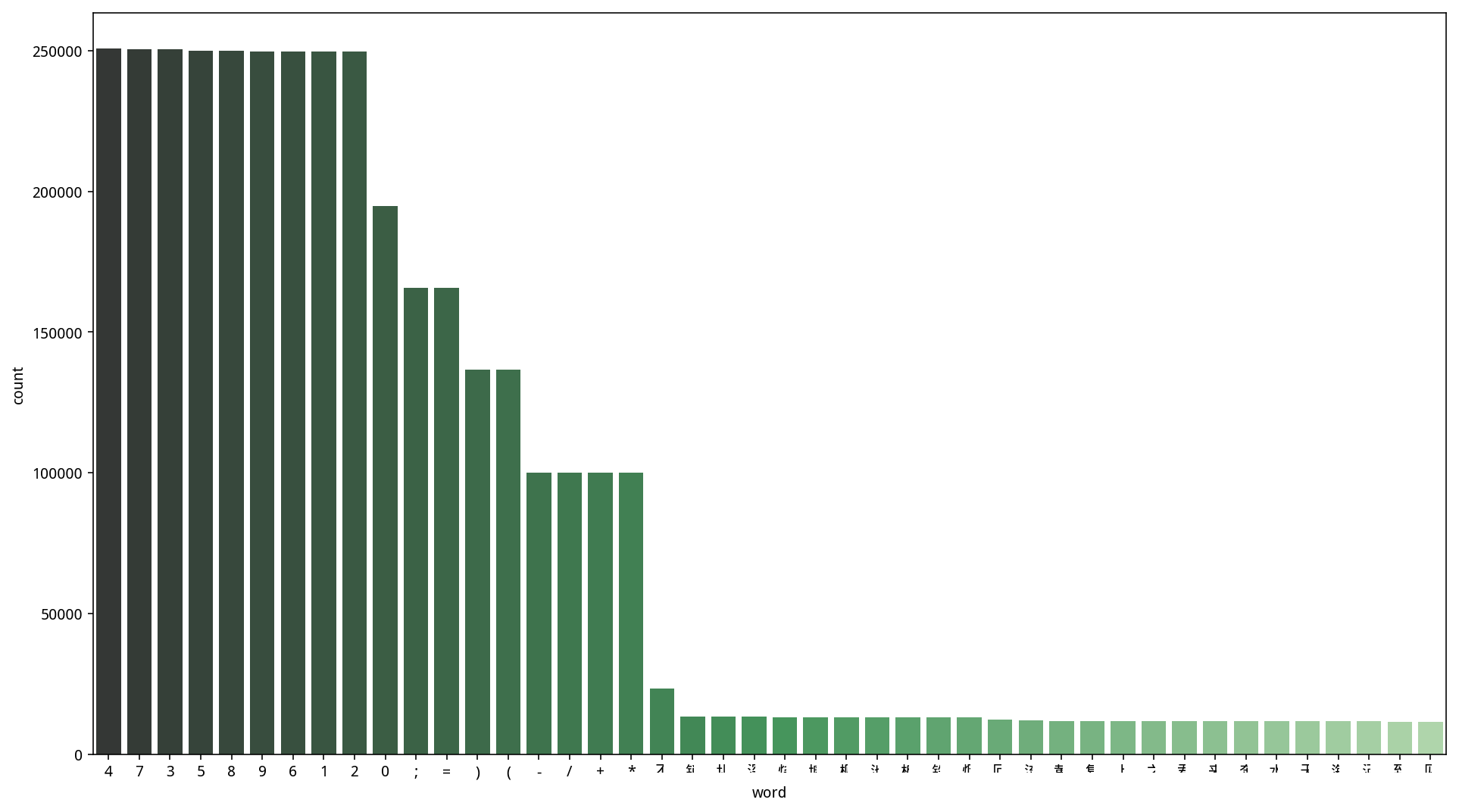``````1+1+1+1

(1+1)+1+1
1+(1+1)+1
1+1+(1+1)
(1+1+1)+1
1+(1+1+1)

((1+1)+1)+1
(1+(1+1))+1

1+((1+1)+1)
1+(1+(1+1))

(1+1)+(1+1)
``````

### 总结

• 中文直接等概率取，“不”概率加倍
• 括号从11种情况中随机取
• 运算符每次必出四个
• 1/3概率取一个赋值式，2/3概率取2个赋值式
• 运算符/永远都会出现一次，中文在上
• 运算符+-*随机取，概率都是1/3
• 数字取值范围是[0, 100000]

## 数据预处理

```def plot(index):
gray = cv2.cvtColor(img, cv2.COLOR_BGR2GRAY)

eq = cv2.equalizeHist(gray)
b = cv2.medianBlur(eq, 9)

m, n = img.shape[:2]
b2 = cv2.resize(b, (n//4, m//4))

m1 = cv2.morphologyEx(b2, cv2.MORPH_OPEN, np.ones((7, 40)))
m2 = cv2.morphologyEx(m1, cv2.MORPH_CLOSE, np.ones((4, 4)))
_, bw = cv2.threshold(m2, 127, 255, cv2.THRESH_BINARY_INV)

bw = cv2.resize(bw, (n, m))

r = img.copy()
img2, ctrs, hier = cv2.findContours(bw, cv2.RETR_EXTERNAL,
cv2.CHAIN_APPROX_SIMPLE)
for ctr in ctrs:
x, y, w, h = cv2.boundingRect(ctr)
cv2.rectangle(r, (x, y), (x+w, y+h), (0, 255, 0), 10)```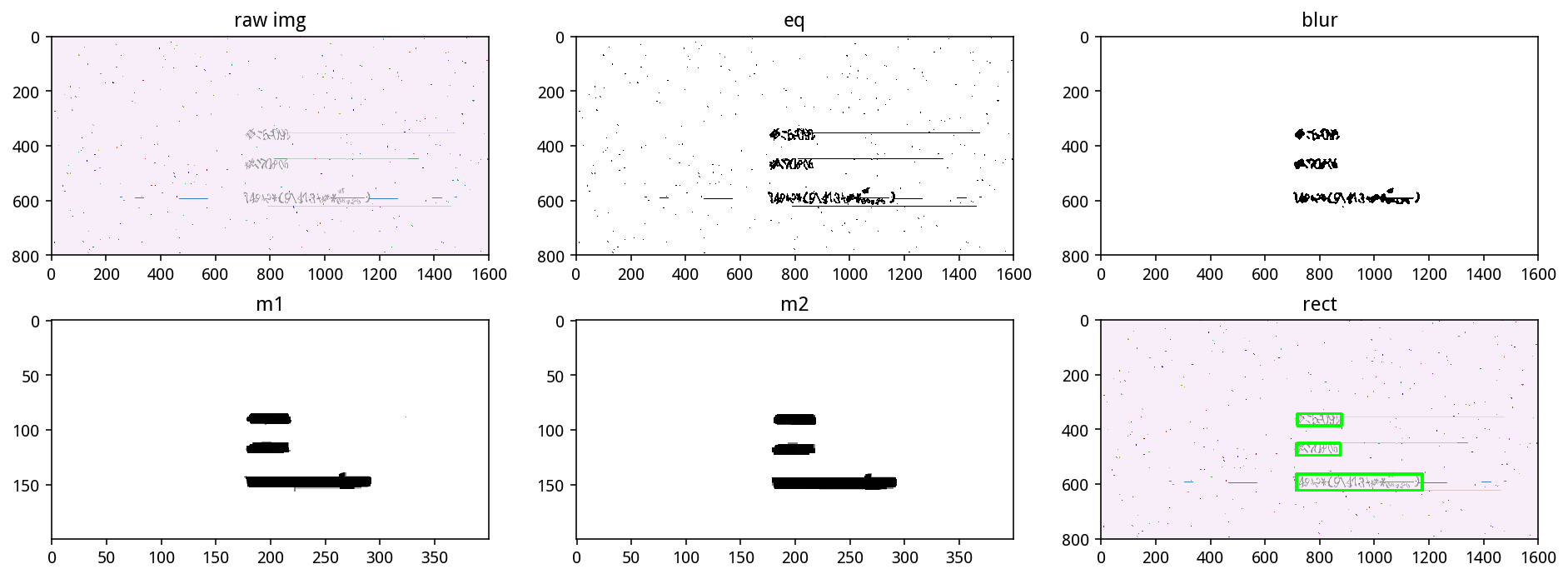### 微调

```# 微调三个公式
d = 20
d2 = 5
imgs = []
sizes = []
for i, ctr in enumerate(ctrs):
x, y, w, h = cv2.boundingRect(ctr)
roi = img[max(0, y-d):min(m, y+h+d),max(0, x-d):min(n, x+w+d)]
p, q, _ = roi.shape

x = b[max(0, y-d):min(m, y+h+d),max(0, x-d):min(n, x+w+d)]
x = cv2.morphologyEx(x, cv2.MORPH_CLOSE, np.ones((3, 3)))
_, x = cv2.threshold(x, 127, 255, cv2.THRESH_BINARY_INV)
_, x, _ = cv2.findContours(x, cv2.RETR_EXTERNAL, cv2.CHAIN_APPROX_SIMPLE)
x, y, w, h = cv2.boundingRect(np.vstack(x))
roi2 = roi[max(0, y-d2):min(p, y+h+d2),max(0, x-d2):min(q, x+w+d2)]
imgs.append(roi2)
sizes.append(roi2.shape)```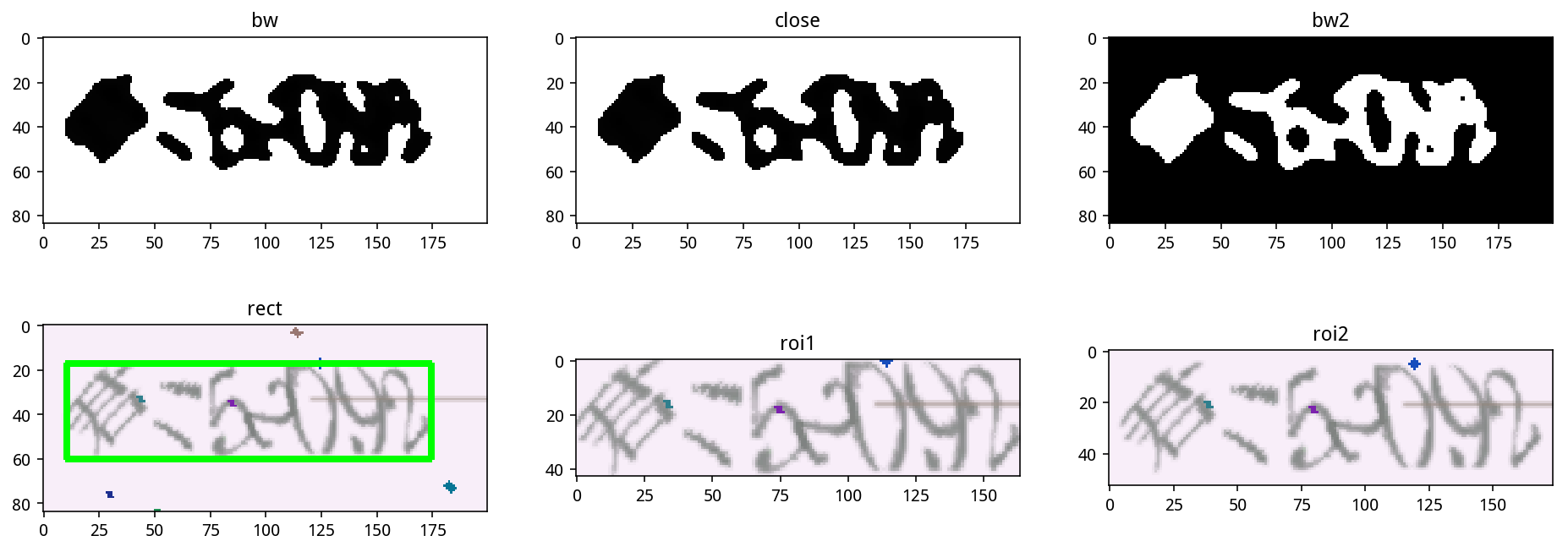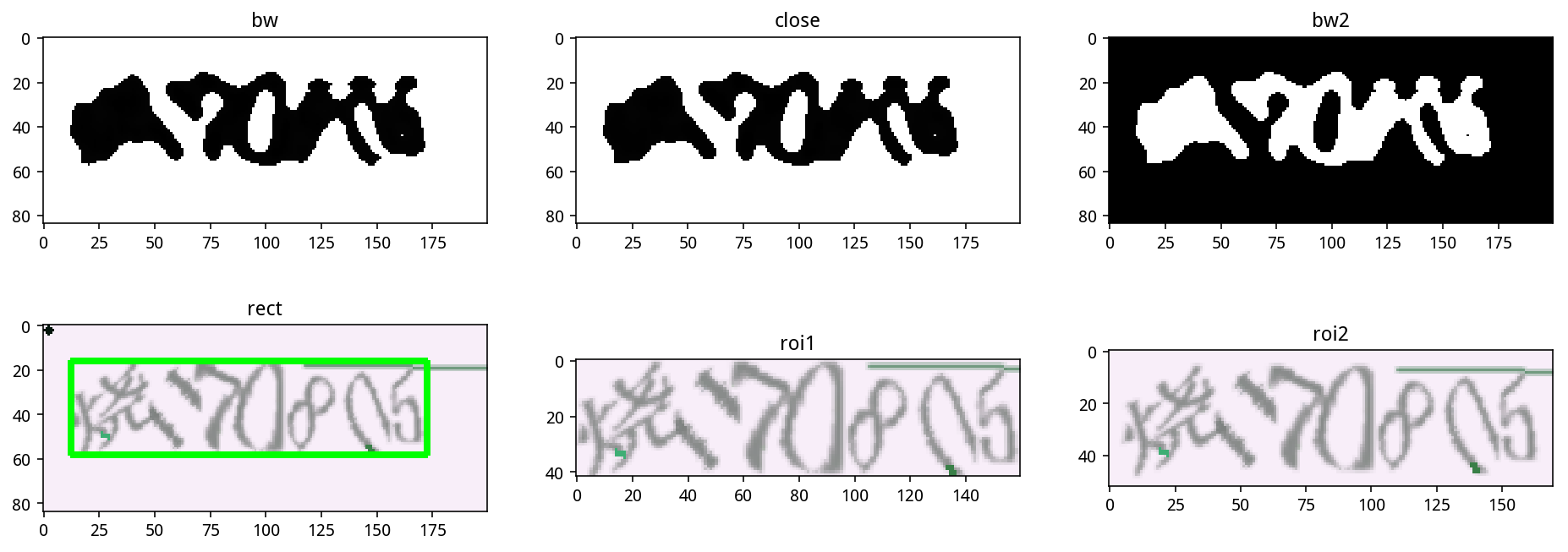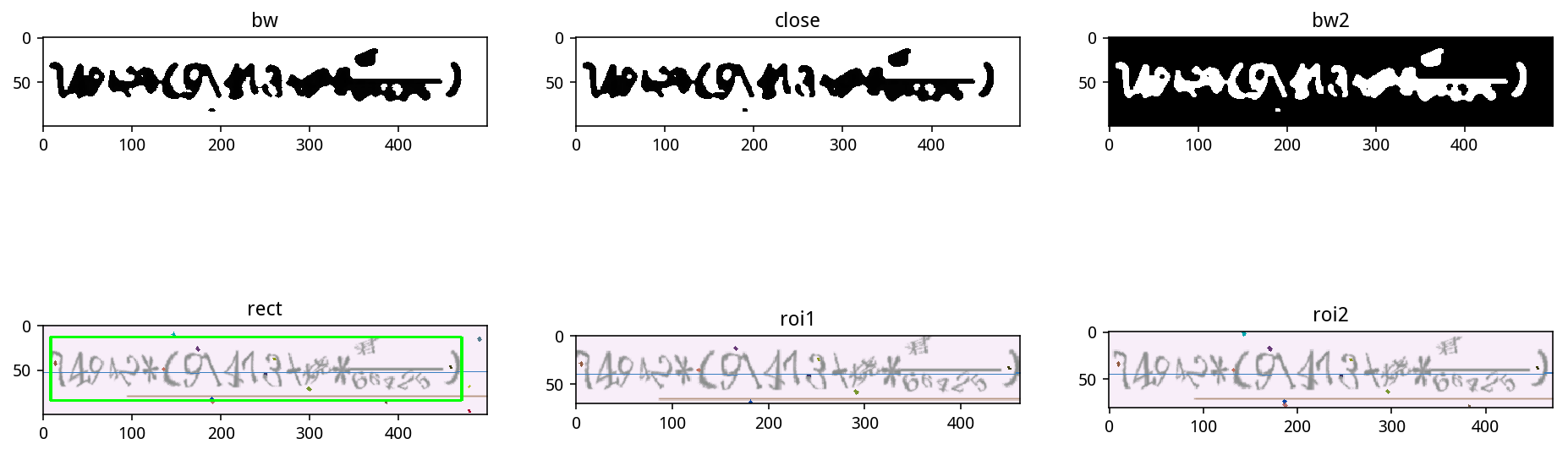### 连接三个公式

```# 连接三个公式
sizes = np.array(sizes)
img2 = np.zeros((sizes[:,0].max(), sizes[:,1].sum()+2*(len(sizes)-1), 3),
dtype=np.uint8)
x = 0
for a in imgs[::-1]:
w = a.shape
img2[:a.shape, x:x+w] = a
x += w + 2```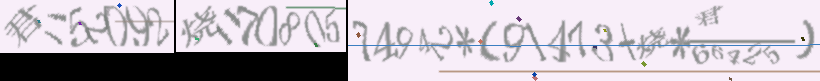### 并行预处理

```p = Pool(12)

n = 100000
if __name__ == '__main__':
rs = []
for r in tqdm(p.imap_unordered(f, range(n)), total=n):
rs.append(r)```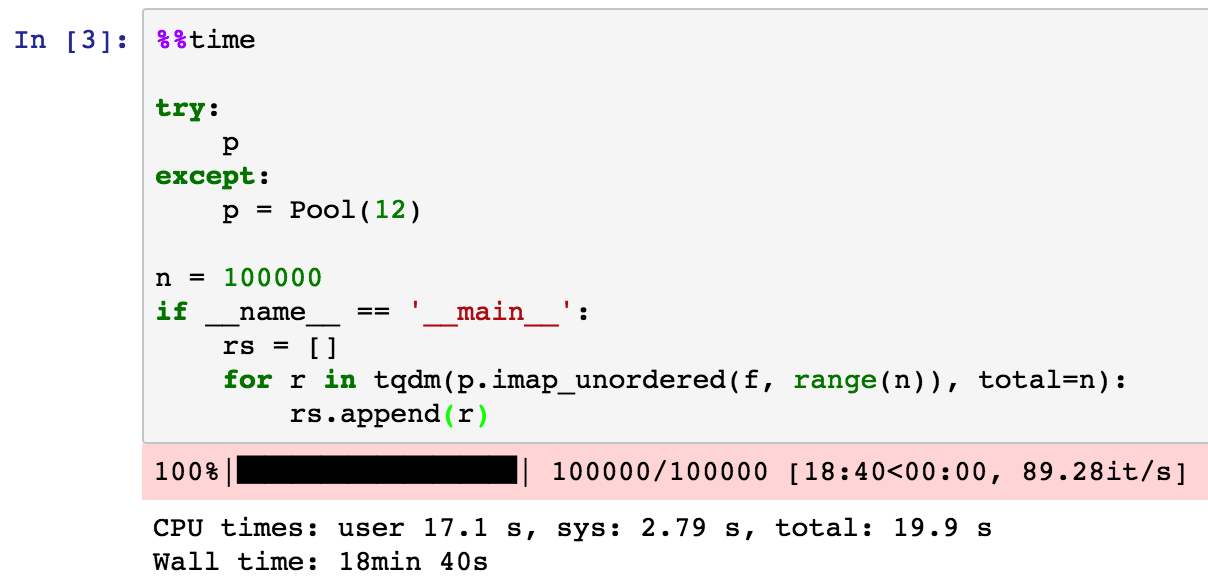### 总结

`pd.plotting.scatter_matrix(df, alpha=0.1, figsize=(14,8), diagonal='kde');`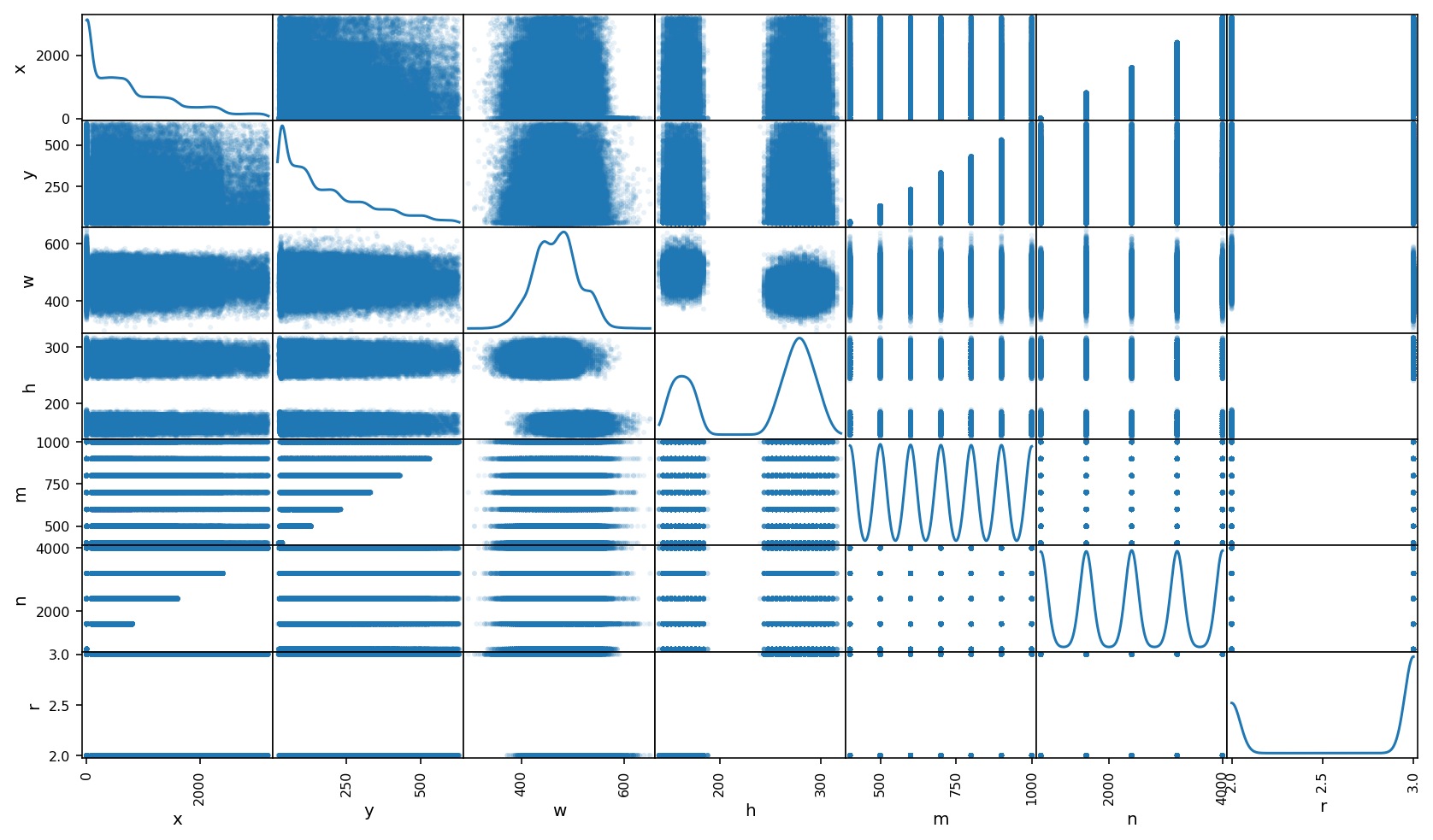w 也没有明显的规律，是典型的正态分布，而 h 则有两个峰，这是因为公式有两个和三个的差别。

m, n 很有规律，它们是按某几个固定的数随机取的，m 的取值是从 [400, 500, 600, 700, 800, 900, 1000] 中随机选取的，n 是从 [800, 1600, 2400, 3200, 4000] 中随机取的。

```Counter(df['m'])
Counter({400: 14233,
500: 14414,
600: 14332,
700: 14304,
800: 14293,
900: 14299,
1000: 14125})

Counter(df['n'])
Counter({800: 19872, 1600: 19937, 2400: 20128, 3200: 19975, 4000: 20088})```

## 模型结构

```def ctc_lambda_func(args):
y_pred, labels, input_length, label_length = args
y_pred = y_pred[:, 2:, :]
return K.ctc_batch_cost(labels, y_pred, input_length, label_length)

rnn_size = 128
l2_rate = 1e-5

input_tensor = Input((width, height, 3))
x = input_tensor
for i, n_cnn in enumerate([3, 4, 6]):
for j in range(n_cnn):
x = Conv2D(32*2**i, (3, 3), padding='same', kernel_initializer='he_uniform',
kernel_regularizer=l2(l2_rate))(x)
x = BatchNormalization(gamma_regularizer=l2(l2_rate), beta_regularizer=l2(l2_rate))(x)
x = Activation('relu')(x)
x = MaxPooling2D((2, 2))(x)

# x = AveragePooling2D((1, 2))(x)
cnn_model = Model(input_tensor, x, name='cnn')

input_tensor = Input((width, height, 3))
x = cnn_model(input_tensor)

conv_shape = x.get_shape().as_list()
rnn_length = conv_shape
rnn_dimen = conv_shape*conv_shape

print conv_shape, rnn_length, rnn_dimen

x = Reshape(target_shape=(rnn_length, rnn_dimen))(x)
rnn_length -= 2
rnn_imp = 0

x = Dense(rnn_size, kernel_initializer='he_uniform', kernel_regularizer=l2(l2_rate), bias_regularizer=l2(l2_rate))(x)
x = BatchNormalization(gamma_regularizer=l2(l2_rate), beta_regularizer=l2(l2_rate))(x)
x = Activation('relu')(x)
# x = Dropout(0.2)(x)

gru_1 = GRU(rnn_size, implementation=rnn_imp, return_sequences=True, name='gru1')(x)
gru_1b = GRU(rnn_size, implementation=rnn_imp, return_sequences=True, go_backwards=True, name='gru1_b')(x)

gru_2 = GRU(rnn_size, implementation=rnn_imp, return_sequences=True, name='gru2')(gru1_merged)
gru_2b = GRU(rnn_size, implementation=rnn_imp, return_sequences=True, go_backwards=True, name='gru2_b')(gru1_merged)
x = concatenate([gru_2, gru_2b])

# x = Dropout(0.2)(x)
x = Dense(n_class, activation='softmax', kernel_regularizer=l2(l2_rate), bias_regularizer=l2(l2_rate))(x)
rnn_out = x
base_model = Model(input_tensor, x)```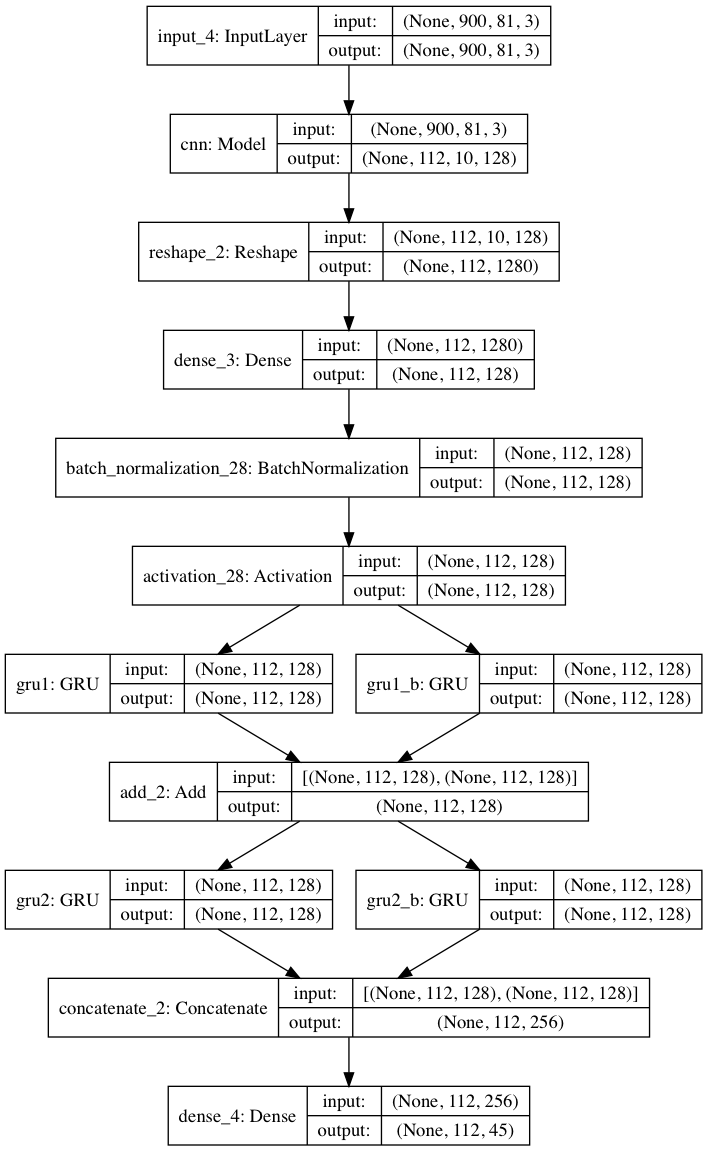### CNN

CNN 的结构如下图：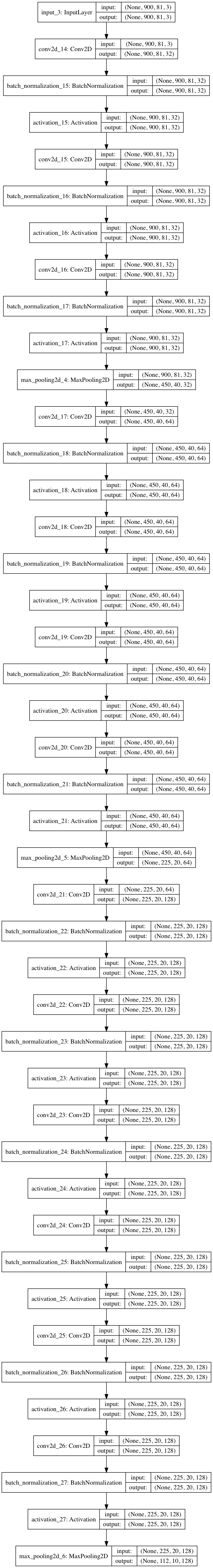CNN 的结构由原来的两层卷积一层池化，改为了多层卷积，一层池化的结构，由于卷积层分别是3，4和6层，我称之为 346 结构。

### GRU

• 前面一个或两个赋值式一定是 中文=数字; 这样的形式
• 左括号一定会有右括号
• 括号的位置是有语法规则的
• 一定会有一个分式
• 分式的分子一定是中文
• 如果只有一个赋值式，那么表达式中的中文一定是赋值式的中文
• 如果有两个赋值式，赋值式容易看清，表达式不容易看清，那么可以通过赋值式的中文去修正表达式的中文，特别是分子中文被裁掉的时候

### 其他参数

• 增加了 l2 正则化，loss loss 变得更大了，但是准确率变得更高了（添加 l2 的部分包括卷积层的 kernel，BN 层的 gamma 和 beta，以及全连接层的 weights 和 bias）
• 各个层的初始化变为了 he_uniform，效果比之前好
• 去掉了 dropout，不清楚影响如何，但是反正有生成器，应该不会出现过拟合的情况
• 修改过 GRU 的 implementation 为2，原因是希望显卡能加速 GRU 的速度，但是似乎速度还不如设置为0，使用 CPU 来跑，所以又改回来了

l2 正则化的参数直接参考了 Xception 论文的 4.3 节给的参数：

Weight decay: The Inception V3 model uses a weight decay (L2 regularization) rate of 4e-5, which has been carefully tuned for performance on ImageNet. We found this rate to be quite suboptimal for Xception and instead settled for 1e-5.

## 生成器

```from collections import defaultdict

cn_imgs = defaultdict(list)
cn_labels = defaultdict(list)
ss_imgs = []
ss_labels = []

for i in tqdm(range(n1)):
ss = df[i].decode('utf-8').split(';')
m = len(ss)-1
ss_labels.append(ss[-1])
for j in range(m):
cn_labels[ss[j]].append(ss[j])

```from keras.utils import Sequence

class SGen(Sequence):
def __init__(self, batch_size):
self.batch_size = batch_size
self.X_gen = np.zeros((batch_size, width, height, 3), dtype=np.uint8)
self.y_gen = np.zeros((batch_size, n_len), dtype=np.uint8)
self.input_length = np.ones(batch_size)*rnn_length
self.label_length = np.ones(batch_size)*38

def __len__(self):
return 350*256 // self.batch_size

def __getitem__(self, idx):
self.X_gen[:] = 0
for i in range(self.batch_size):
try:
random_index = random.randint(0, n1-1)
cls = []
ss = ss_labels[random_index]
cs = re.findall(ur'[\u4e00-\u9fff]', df[random_index].decode('utf-8').split(';')[-1])
random.shuffle(cs)
x = 0
for c in cs:
random_index2 = random.randint(0, len(cn_labels[c])-1)
cls.append(cn_labels[c][random_index2])
img = cn_imgs[c][random_index2]
w, h, _ = img.shape
self.X_gen[i, x:x+w, :h] = img
x += w+2
img = ss_imgs[random_index]
w, h, _ = img.shape
self.X_gen[i, x:x+w, :h] = img
cls.append(ss)

random_str = u';'.join(cls)
self.y_gen[i,:len(random_str)] = [characters.find(x) for x in random_str]
self.y_gen[i,len(random_str):] = n_class-1
self.label_length[i] = len(random_str)
except:
pass

return [self.X_gen, self.y_gen, self.input_length, self.label_length], np.ones(self.batch_size)```## 训练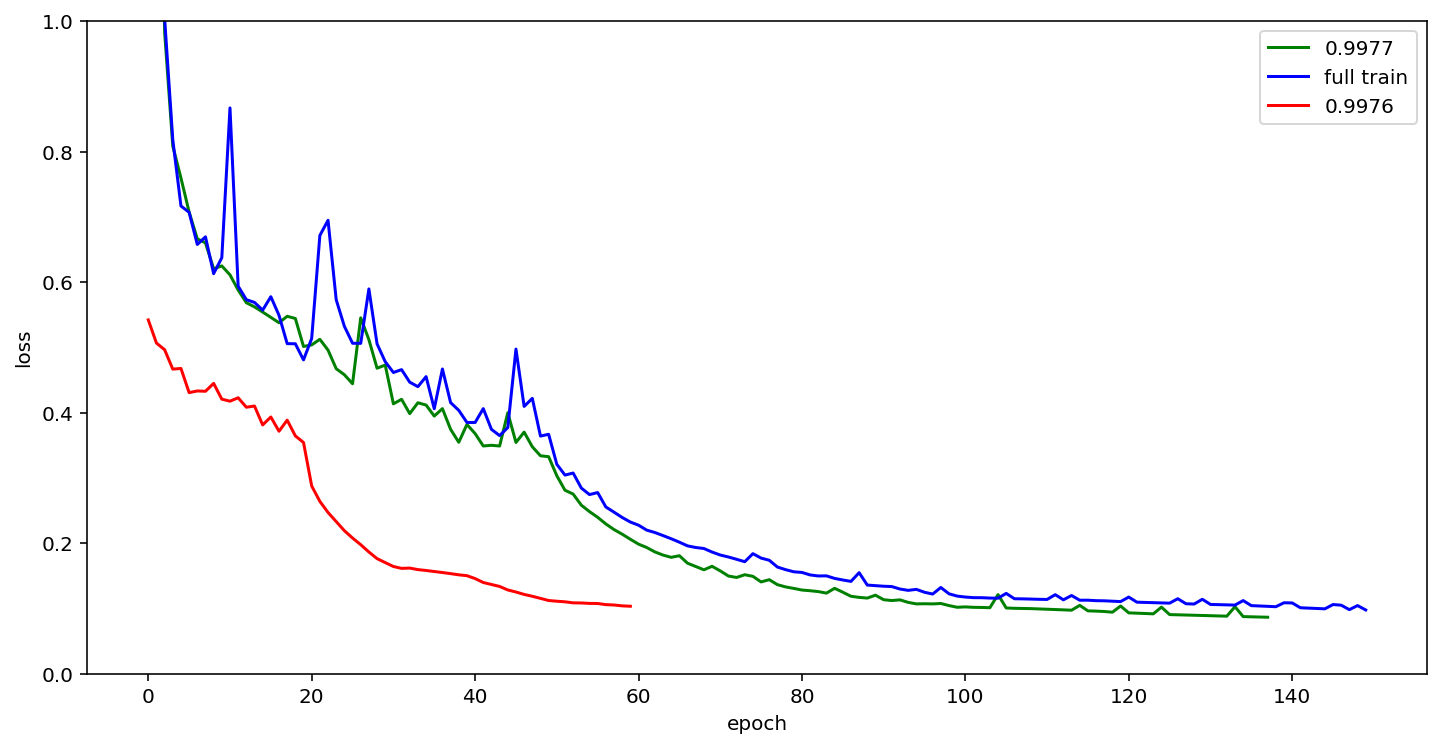## 预测结果

```X = np.zeros((n, width, height, channels), dtype=np.uint8)

for i in tqdm(range(n)):
a, b, _ = img.shape
X[i, :a, :b] = img

base_model2 = make_parallel(base_model, 4)

y_pred = base_model2.predict(X, batch_size=500, verbose=1)
out = K.get_value(K.ctc_decode(y_pred[:,2:], input_length=np.ones(y_pred.shape)*rnn_length))[:, :n_len]```

```ss = map(decode, out)

vals = []
errs = []
errsid = []
for i in tqdm(range(100000)):
val = ''
try:
a = ss[i].split(';')
s = a[-1]
for x in a[:-1]:
x, c = x.split('=')
s = s.replace(x, c+'.0')
val = '%.2f' % eval(s)
except:
#         disp3(i)
errs.append(ss[i])
errsid.append(i)
ss[i] = ''

vals.append(val)

with open('result_%s.txt' % z, 'w') as f:
f.write('\n'.join(map(' '.join, list(zip(ss, vals)))).encode('utf-8'))

print len(errs)
print 1-len(errs)/100000.

# output
22
0.99978```

## 模型结果融合

```import glob
import numpy as np
from collections import Counter

def fun(x):
c = Counter(x)
c[' '] = 0
return c.most_common()

ss = [open(fname, 'r').read().split('\n') for fname in glob.glob('result_model*.txt')]
s = np.array(ss).T
with open('result.txt', 'w') as f:
f.write('\n'.join(map(fun, s)))```

## 其他尝试

### 生成器尝试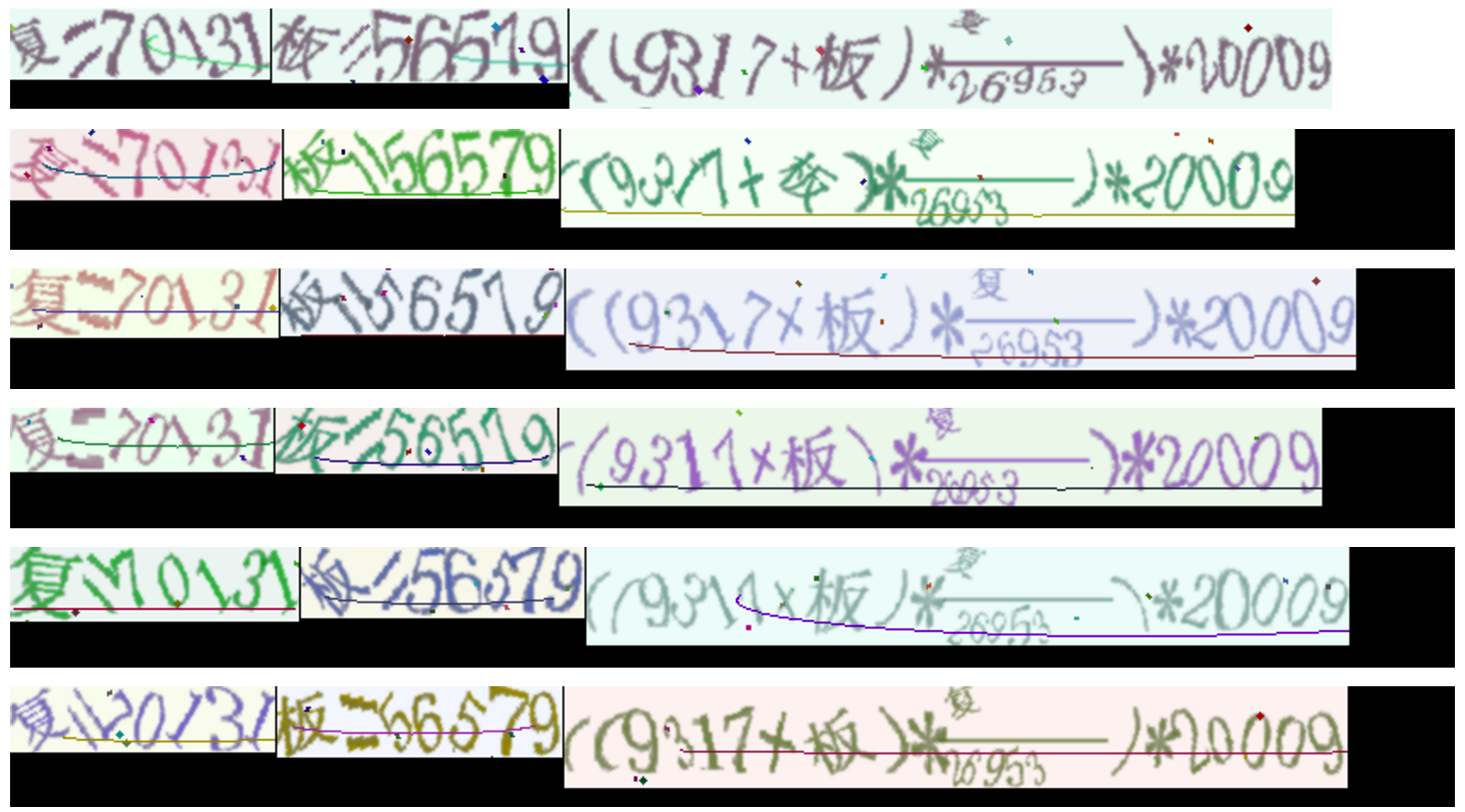### 其他 CNN 模型的尝试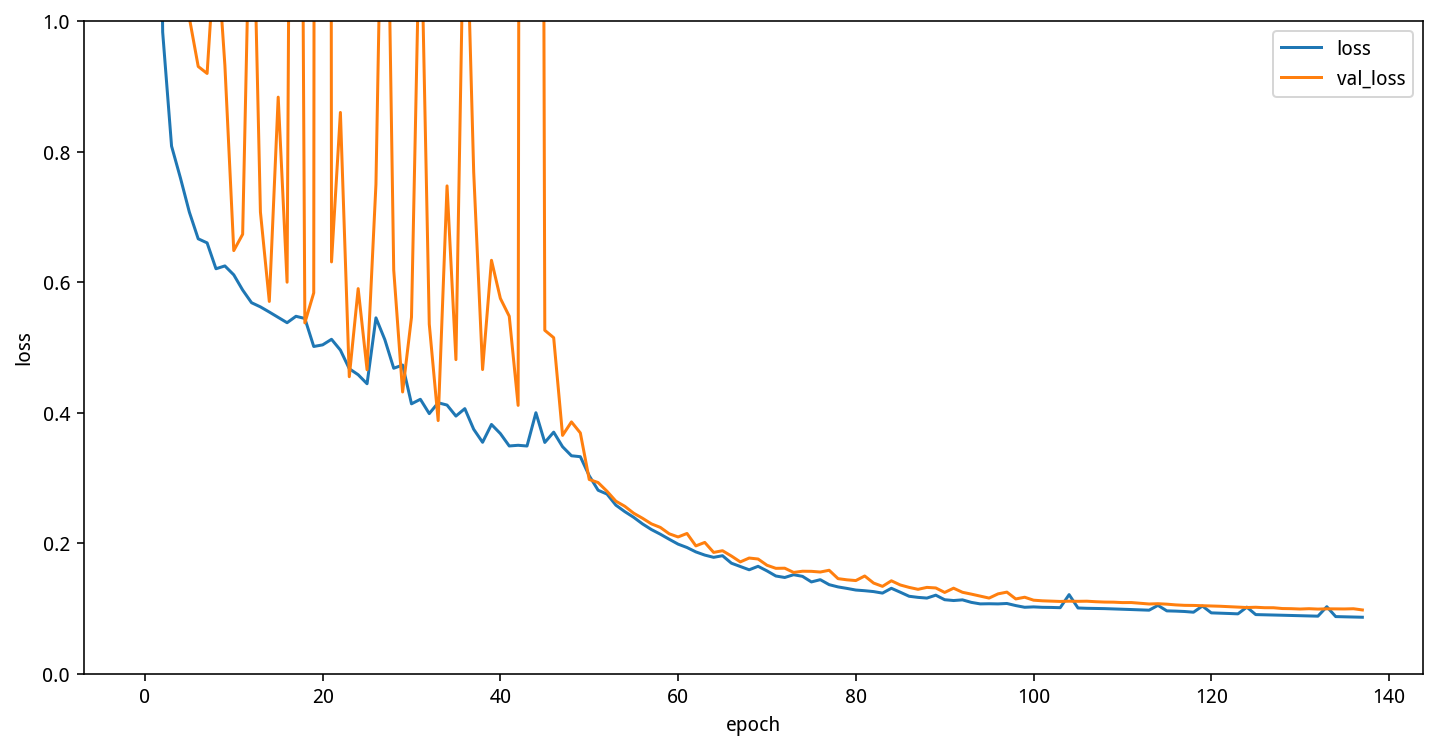### 替换 GRU 为 LSTM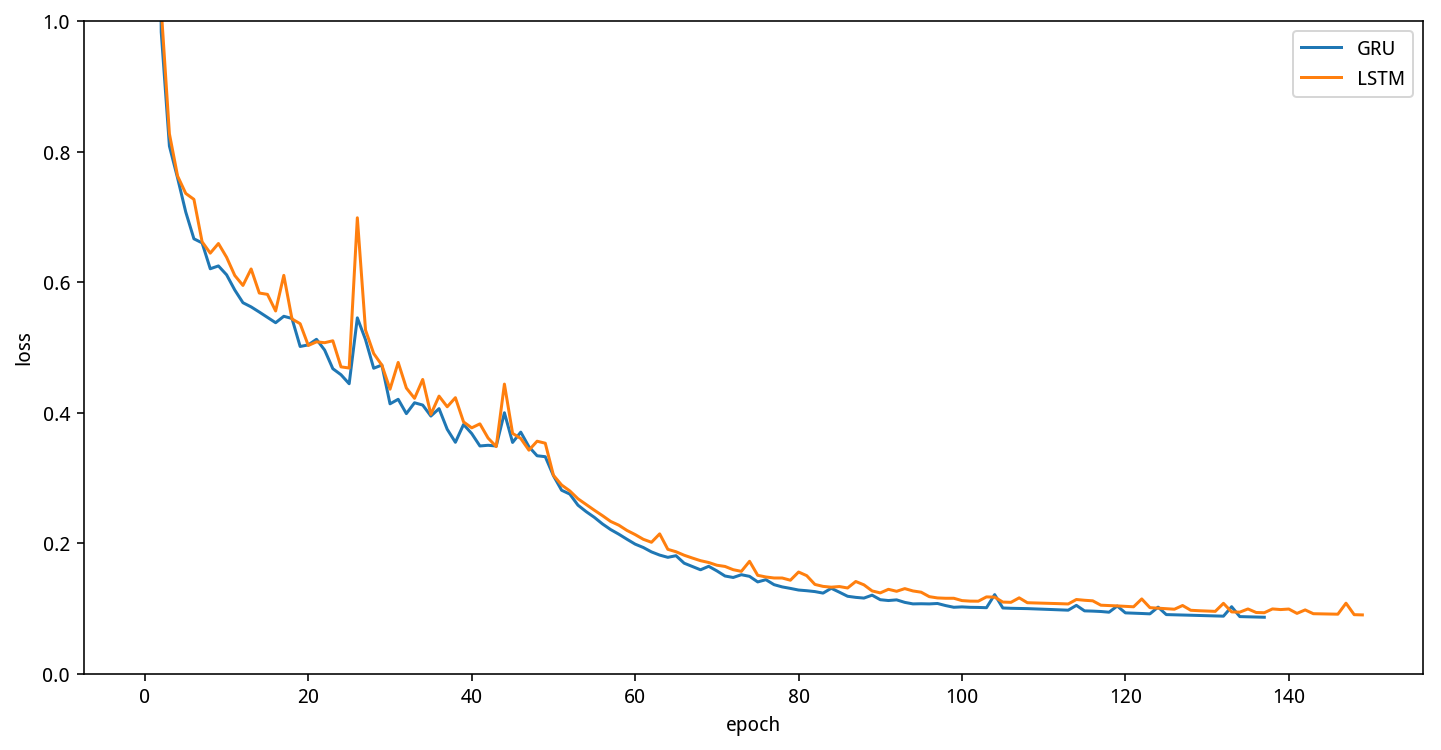## 总结

### 对项目的思考

• 数据准备：
• 深度学习同传统图像处理技术结合，可以达到更好的准确率
• 文本识别可以构造验证码生成器进行数据增强，增加训练样本数
• 模型优化：
• 如何根据项目特点，对模型结构进行调整，如CNN 部分减少池化层使用，等等
• 为了防止过拟合，在模型中引入 L2 正则化
• 模型训练：
• 使用学习率衰减策略，训练模型
• 对复杂的模型，可以将同一批次输入数据分摊给多个GPU进行计算。

### 有趣的样本

#### 95170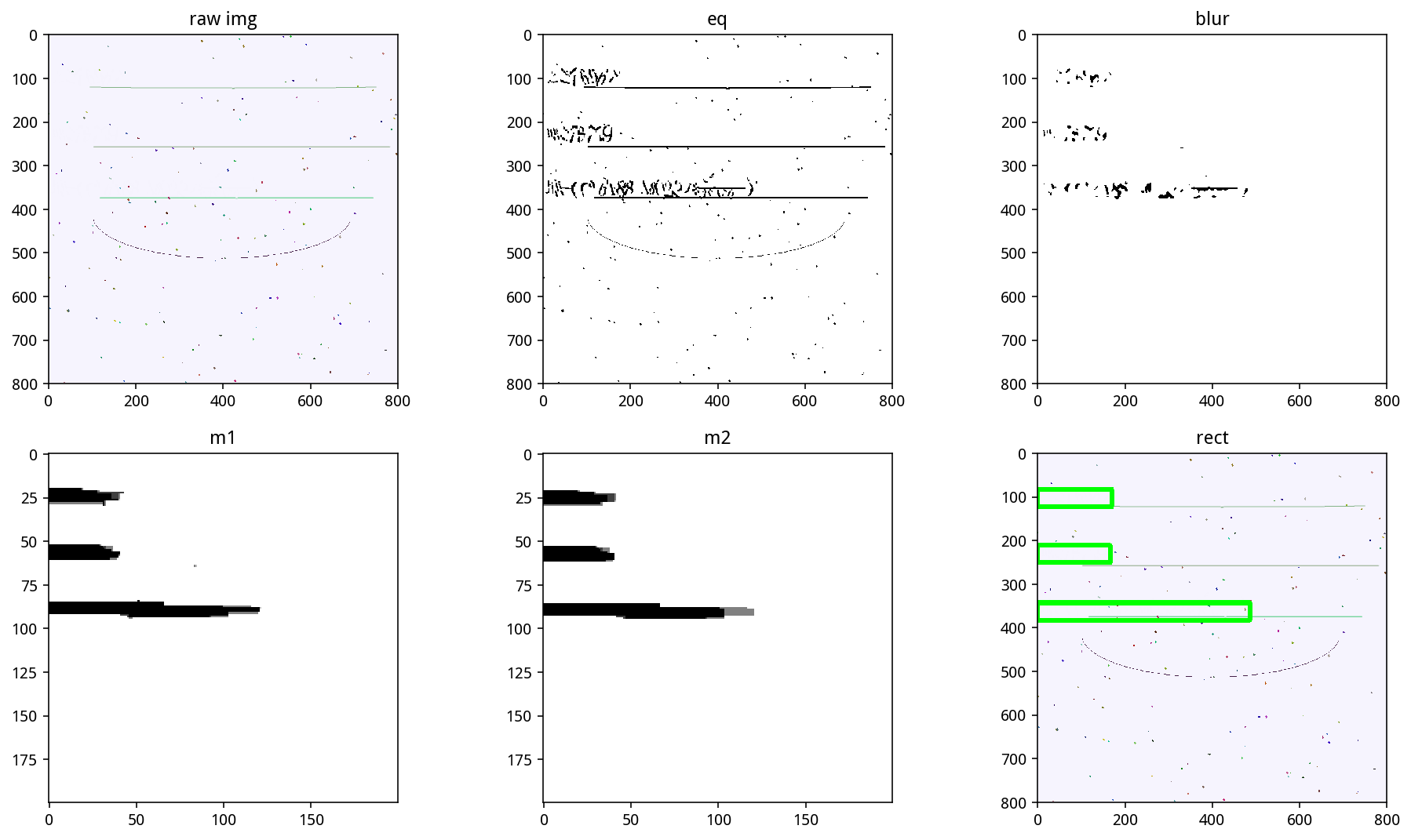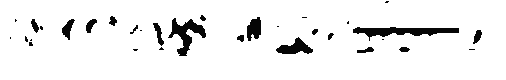#### 干扰线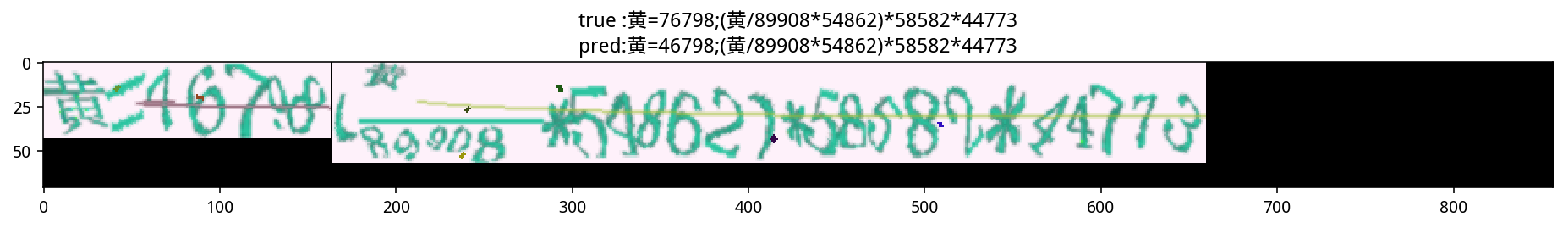### 可能的改进

• 将真正的生成器写出来，这样就可以获取无穷无尽的样本，而不是使用已有的样本进行拼接，对5和6的识别，以及很多横向的中文的识别，会有很好的帮助，因为它们在已有样本中十分罕见，以至于模型无法准确分辨5和6，以及横向的中文
• 做更好的预处理，比如干扰线和字的颜色是不同的，可以通过程序去除，切图可以更精准一些，可以极大提高训练速度
• 使用其他的模型，比如群里有人提到的 attention 模型，或者看看 OCR 相关的论文，找更多的模型，融合结果，比直接跑类似结构的模型来融合的效果会好很多